Скачать презентацию CHAPTER 8 Risk and Rates of Return n

556b48524e3e0b9d4e364ccf2eb29374.ppt

• Количество слайдов: 54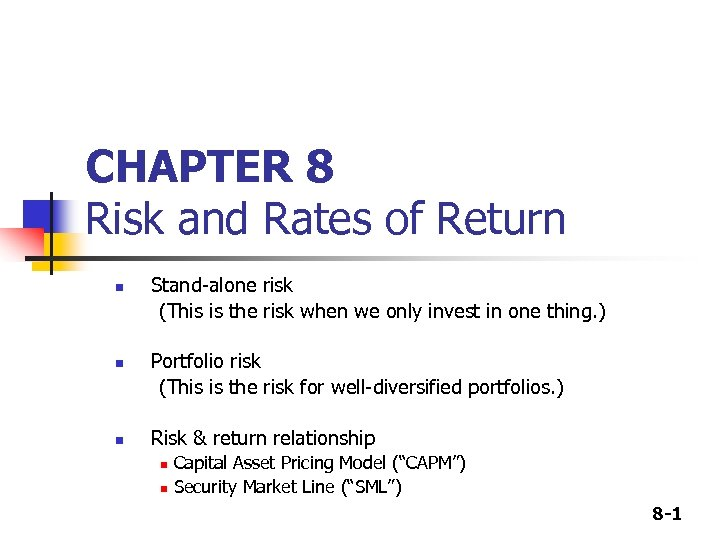CHAPTER 8 Risk and Rates of Return n Stand-alone risk (This is the risk when we only invest in one thing. ) Portfolio risk (This is the risk for well-diversified portfolios. ) Risk & return relationship n n Capital Asset Pricing Model (“CAPM”) Security Market Line (“SML”) 8 -1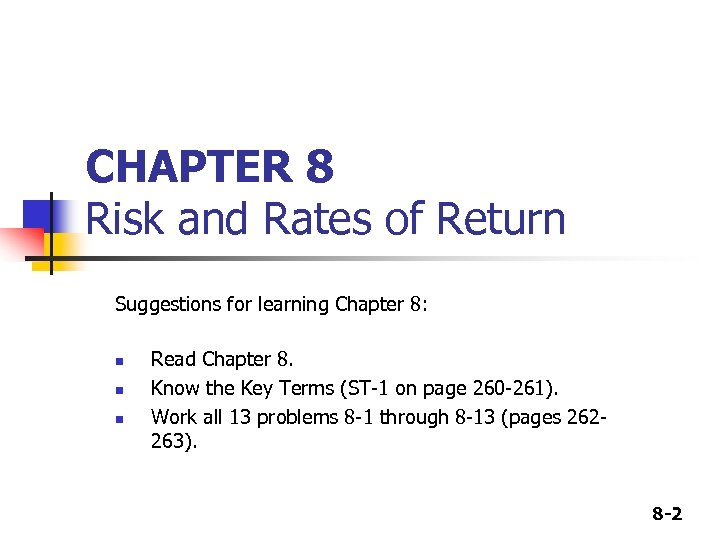CHAPTER 8 Risk and Rates of Return Suggestions for learning Chapter 8: n n n Read Chapter 8. Know the Key Terms (ST-1 on page 260 -261). Work all 13 problems 8 -1 through 8 -13 (pages 262263). 8 -2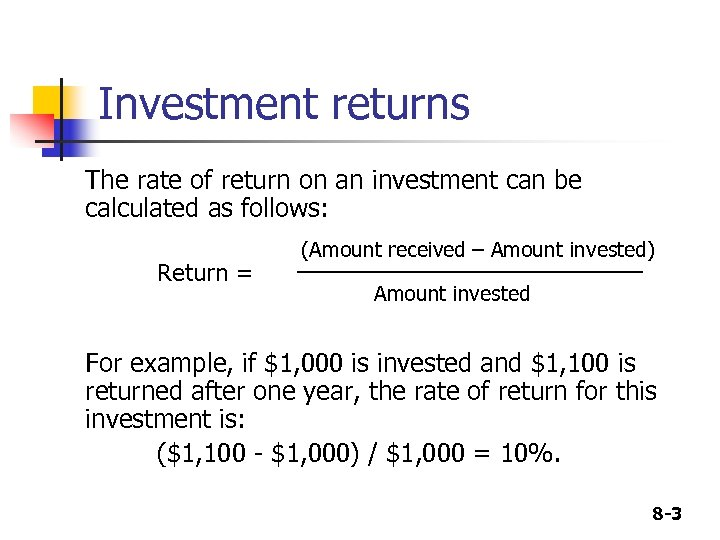Investment returns The rate of return on an investment can be calculated as follows: Return = (Amount received – Amount invested) ____________ Amount invested For example, if \$1, 000 is invested and \$1, 100 is returned after one year, the rate of return for this investment is: (\$1, 100 - \$1, 000) / \$1, 000 = 10%. 8 -3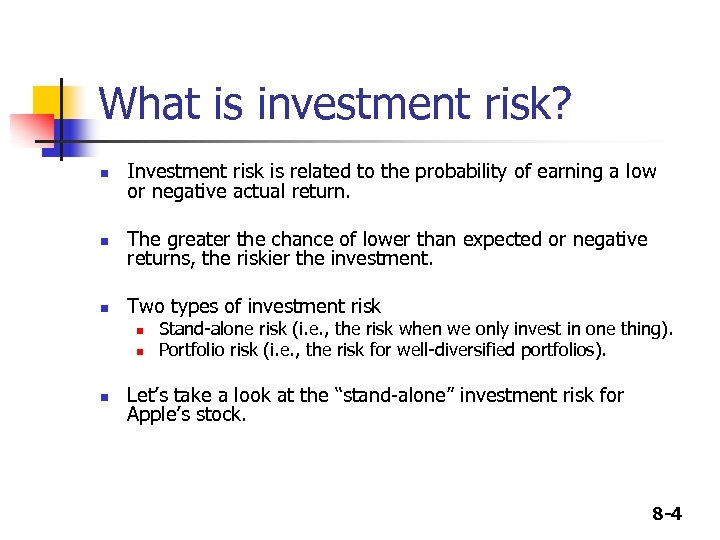What is investment risk? n Investment risk is related to the probability of earning a low or negative actual return. n The greater the chance of lower than expected or negative returns, the riskier the investment. n Two types of investment risk n n n Stand-alone risk (i. e. , the risk when we only invest in one thing). Portfolio risk (i. e. , the risk for well-diversified portfolios). Let’s take a look at the “stand-alone” investment risk for Apple’s stock. 8 -4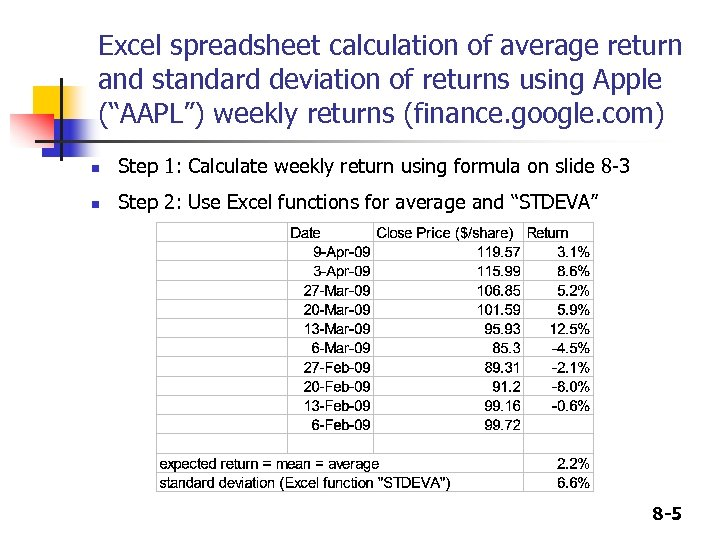Excel spreadsheet calculation of average return and standard deviation of returns using Apple (“AAPL”) weekly returns (finance. google. com) n Step 1: Calculate weekly return using formula on slide 8 -3 n Step 2: Use Excel functions for average and “STDEVA” 8 -5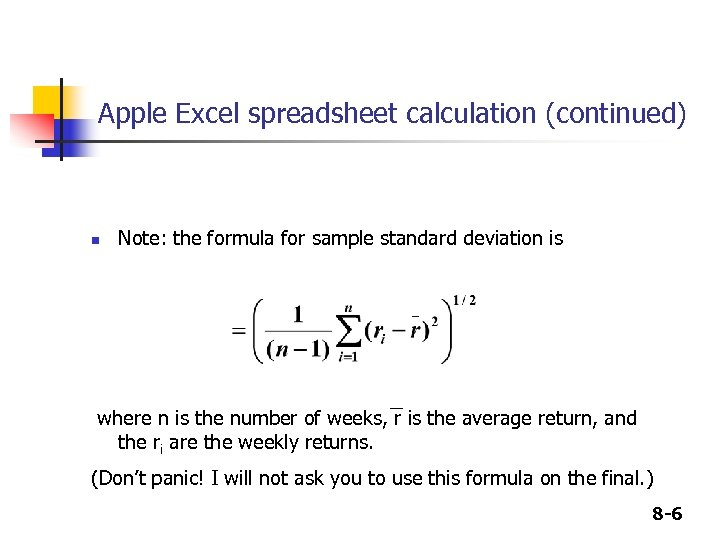Apple Excel spreadsheet calculation (continued) n Note: the formula for sample standard deviation is where n is the number of weeks, r is the average return, and the ri are the weekly returns. (Don’t panic! I will not ask you to use this formula on the final. ) 8 -6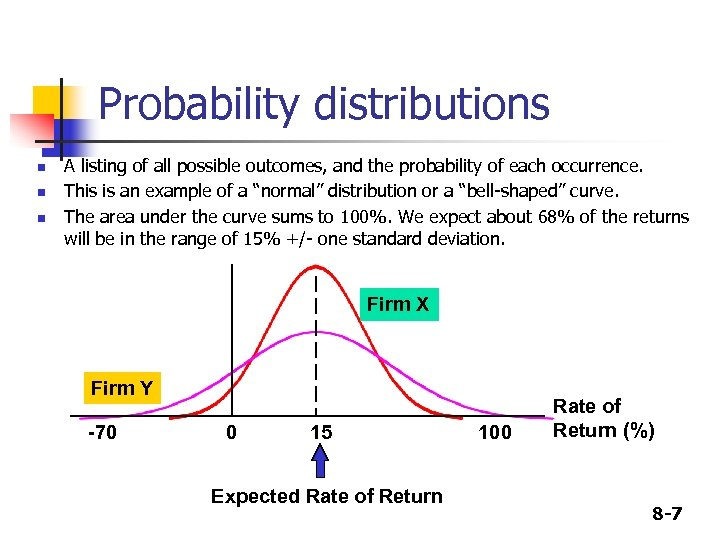Probability distributions n n n A listing of all possible outcomes, and the probability of each occurrence. This is an example of a “normal” distribution or a “bell-shaped” curve. The area under the curve sums to 100%. We expect about 68% of the returns will be in the range of 15% +/- one standard deviation. Firm X Firm Y -70 0 15 Expected Rate of Return 100 Rate of Return (%) 8 -7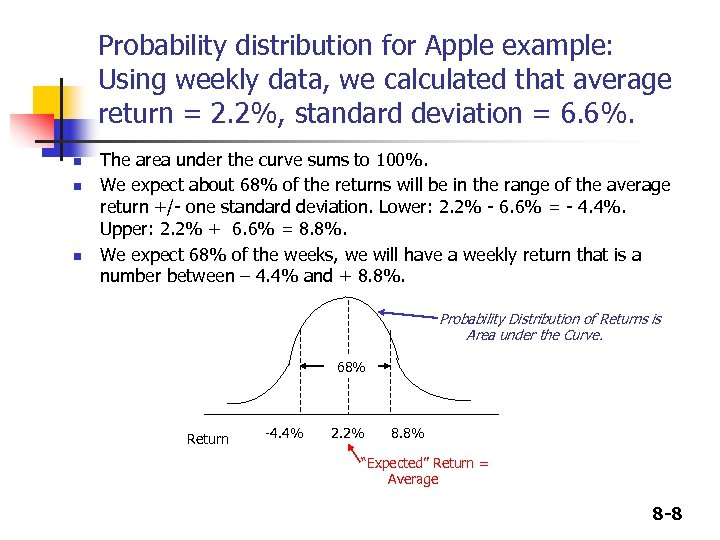Probability distribution for Apple example: Using weekly data, we calculated that average return = 2. 2%, standard deviation = 6. 6%. n n n The area under the curve sums to 100%. We expect about 68% of the returns will be in the range of the average return +/- one standard deviation. Lower: 2. 2% - 6. 6% = - 4. 4%. Upper: 2. 2% + 6. 6% = 8. 8%. We expect 68% of the weeks, we will have a weekly return that is a number between – 4. 4% and + 8. 8%. Probability Distribution of Returns is Area under the Curve. 68% Return -4. 4% 2. 2% 8. 8% “Expected” Return = Average 8 -8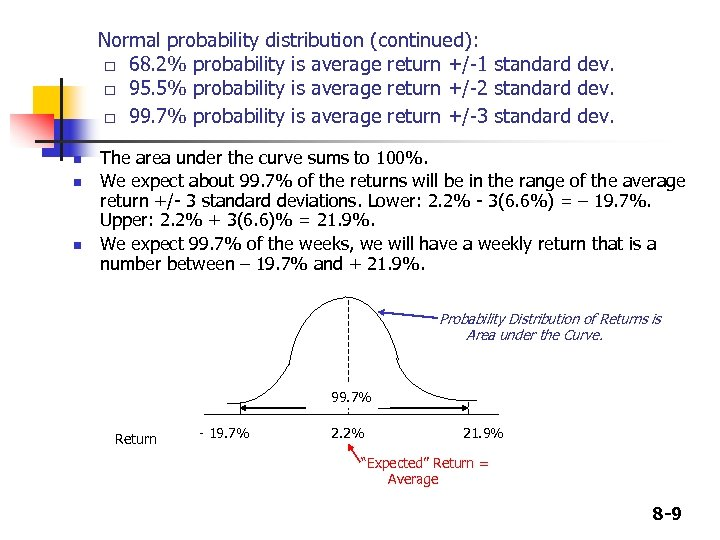Normal probability distribution (continued): □ 68. 2% probability is average return +/-1 standard dev. □ 95. 5% probability is average return +/-2 standard dev. □ 99. 7% probability is average return +/-3 standard dev. n n n The area under the curve sums to 100%. We expect about 99. 7% of the returns will be in the range of the average return +/- 3 standard deviations. Lower: 2. 2% - 3(6. 6%) = – 19. 7%. Upper: 2. 2% + 3(6. 6)% = 21. 9%. We expect 99. 7% of the weeks, we will have a weekly return that is a number between – 19. 7% and + 21. 9%. Probability Distribution of Returns is Area under the Curve. 99. 7% Return - 19. 7% 2. 2% 21. 9% “Expected” Return = Average 8 -9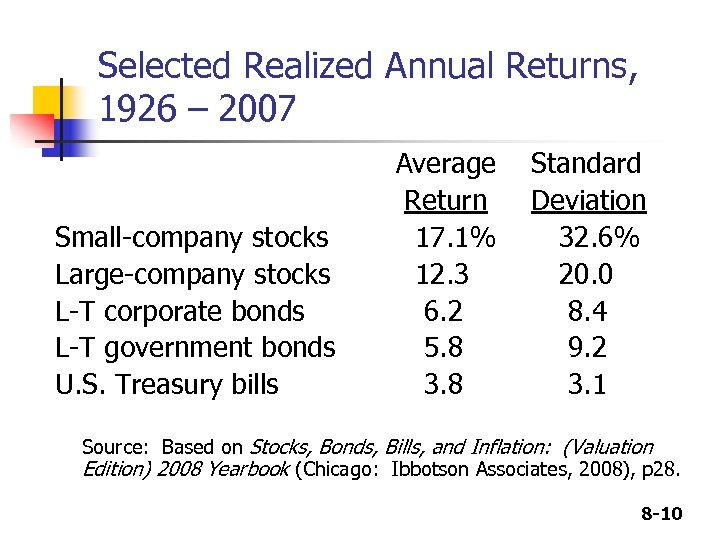Selected Realized Annual Returns, 1926 – 2007 Small-company stocks Large-company stocks L-T corporate bonds L-T government bonds U. S. Treasury bills Average Return 17. 1% 12. 3 6. 2 5. 8 3. 8 Standard Deviation 32. 6% 20. 0 8. 4 9. 2 3. 1 Source: Based on Stocks, Bonds, Bills, and Inflation: (Valuation Edition) 2008 Yearbook (Chicago: Ibbotson Associates, 2008), p 28. 8 -10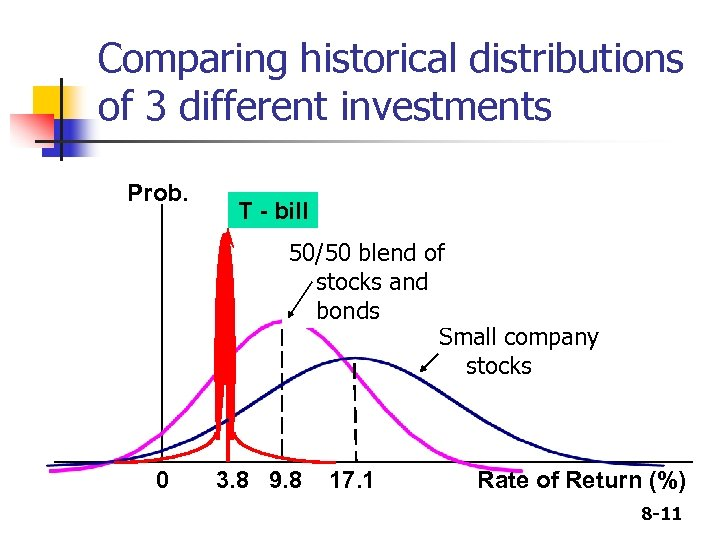Comparing historical distributions of 3 different investments Prob. T - bill 50/50 blend of stocks USR and bonds Small company HT stocks 0 3. 8 9. 8 17. 1 Rate of Return (%) 8 -11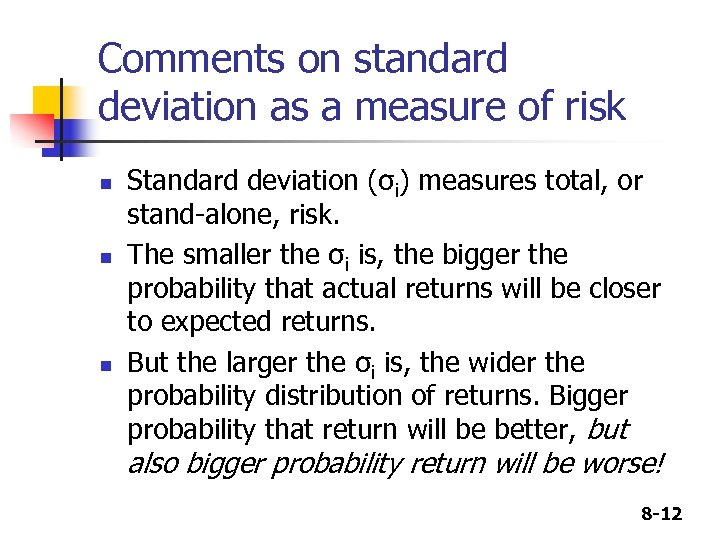Comments on standard deviation as a measure of risk n n n Standard deviation (σi) measures total, or stand-alone, risk. The smaller the σi is, the bigger the probability that actual returns will be closer to expected returns. But the larger the σi is, the wider the probability distribution of returns. Bigger probability that return will be better, but also bigger probability return will be worse! 8 -12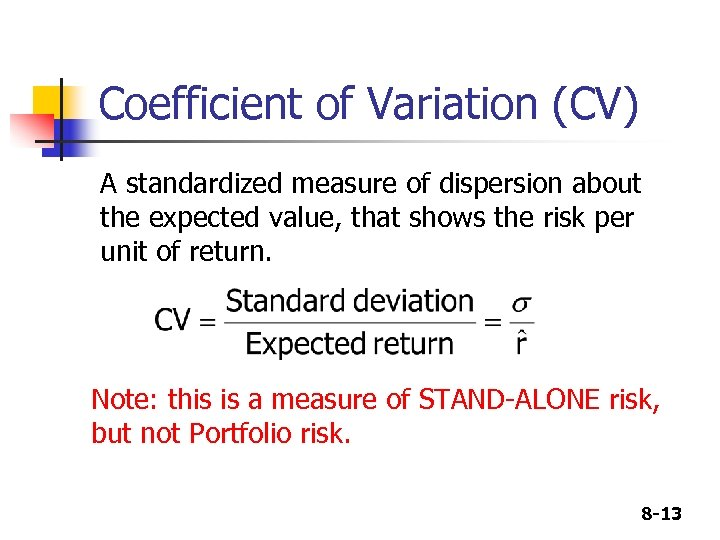Coefficient of Variation (CV) A standardized measure of dispersion about the expected value, that shows the risk per unit of return. Note: this is a measure of STAND-ALONE risk, but not Portfolio risk. 8 -13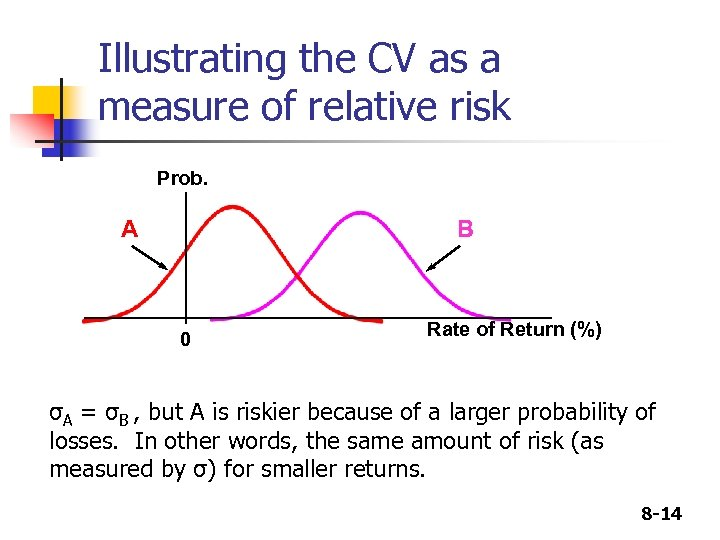Illustrating the CV as a measure of relative risk Prob. A B 0 Rate of Return (%) σA = σB , but A is riskier because of a larger probability of losses. In other words, the same amount of risk (as measured by σ) for smaller returns. 8 -14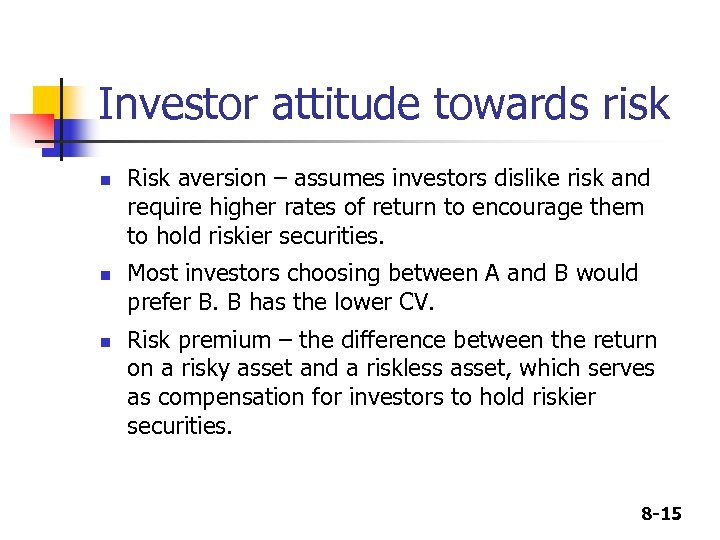Investor attitude towards risk n n n Risk aversion – assumes investors dislike risk and require higher rates of return to encourage them to hold riskier securities. Most investors choosing between A and B would prefer B. B has the lower CV. Risk premium – the difference between the return on a risky asset and a riskless asset, which serves as compensation for investors to hold riskier securities. 8 -15Consider these forecasts for 5 investment alternatives (from Integrated Case, pages 266 -237 of your text) T-Bill High Tech (HT) Collections USR Market Portfolio (MP) 0. 1 5. 5% -27. 0% 6. 0% -17. 0% Below avg 0. 2 5. 5% -7. 0% 13. 0% -14. 0% -3. 0% Average 0. 4 5. 5% 15. 0% 0. 0% 3. 0% 10. 0% Above avg 0. 2 5. 5% 30. 0% -11. 0% 41. 0% 25. 0% Boom 0. 1 1. 0 5. 5% 45. 0% -21. 0% 26. 0% 38. 0% Forecast of Economy Probability Recession 8 -16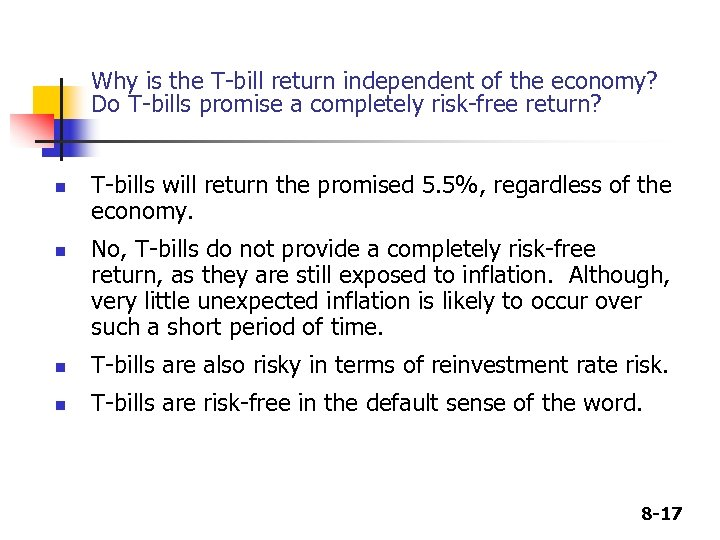Why is the T-bill return independent of the economy? Do T-bills promise a completely risk-free return? n n T-bills will return the promised 5. 5%, regardless of the economy. No, T-bills do not provide a completely risk-free return, as they are still exposed to inflation. Although, very little unexpected inflation is likely to occur over such a short period of time. n T-bills are also risky in terms of reinvestment rate risk. n T-bills are risk-free in the default sense of the word. 8 -17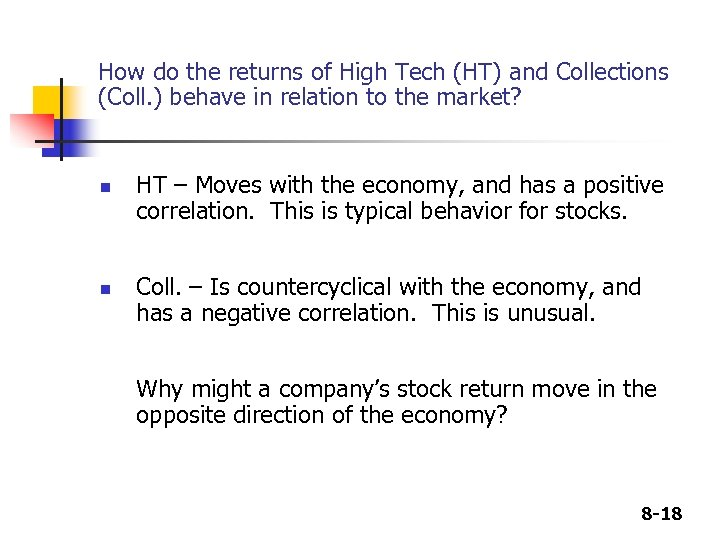How do the returns of High Tech (HT) and Collections (Coll. ) behave in relation to the market? n n HT – Moves with the economy, and has a positive correlation. This is typical behavior for stocks. Coll. – Is countercyclical with the economy, and has a negative correlation. This is unusual. Why might a company’s stock return move in the opposite direction of the economy? 8 -18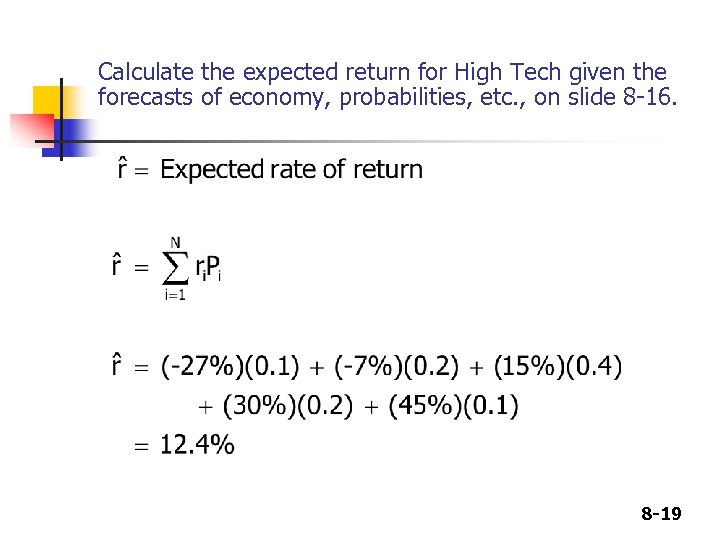Calculate the expected return for High Tech given the forecasts of economy, probabilities, etc. , on slide 8 -16. 8 -19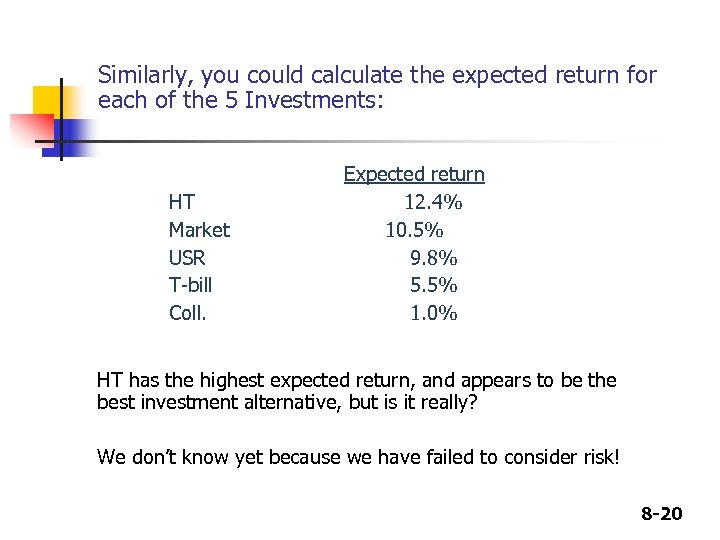Similarly, you could calculate the expected return for each of the 5 Investments: HT Market USR T-bill Coll. Expected return 12. 4% 10. 5% 9. 8% 5. 5% 1. 0% HT has the highest expected return, and appears to be the best investment alternative, but is it really? We don’t know yet because we have failed to consider risk! 8 -20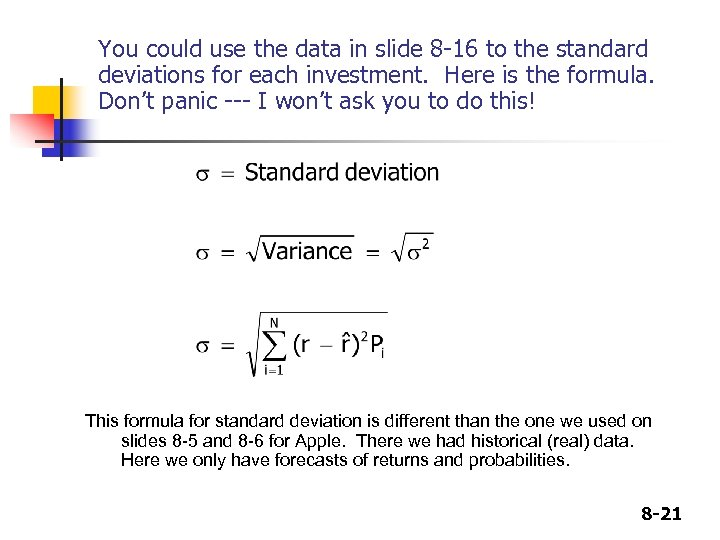You could use the data in slide 8 -16 to the standard deviations for each investment. Here is the formula. Don’t panic --- I won’t ask you to do this! This formula for standard deviation is different than the one we used on slides 8 -5 and 8 -6 for Apple. There we had historical (real) data. Here we only have forecasts of returns and probabilities. 8 -21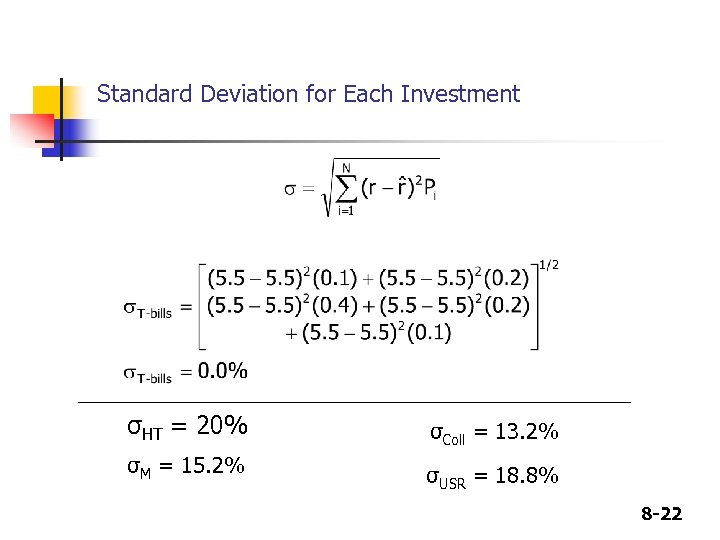Standard Deviation for Each Investment σHT = 20% σColl = 13. 2% σM = 15. 2% σUSR = 18. 8% 8 -22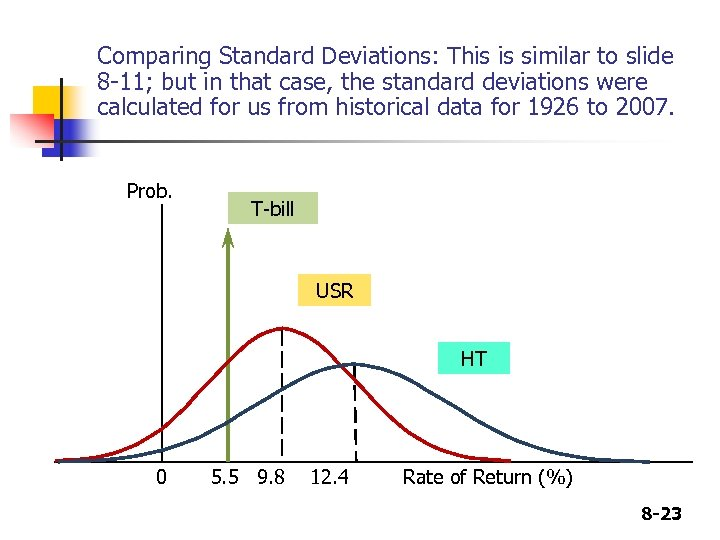Comparing Standard Deviations: This is similar to slide 8 -11; but in that case, the standard deviations were calculated for us from historical data for 1926 to 2007. Prob. T-bill USR HT 0 5. 5 9. 8 12. 4 Rate of Return (%) 8 -23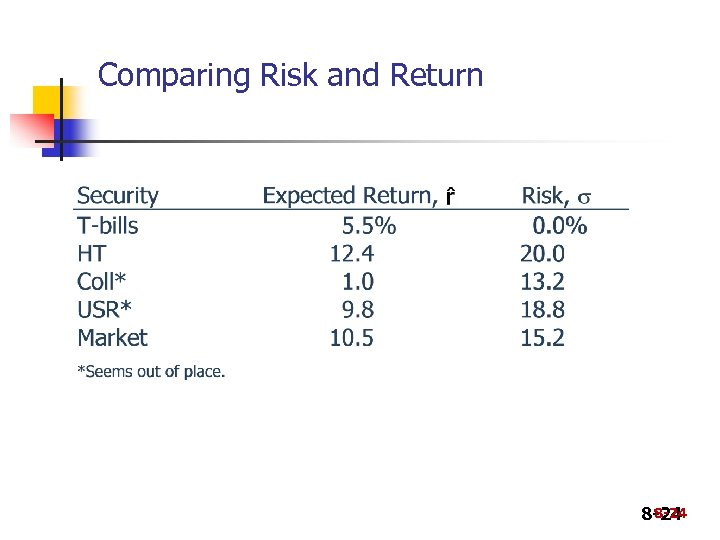Comparing Risk and Return 8 -24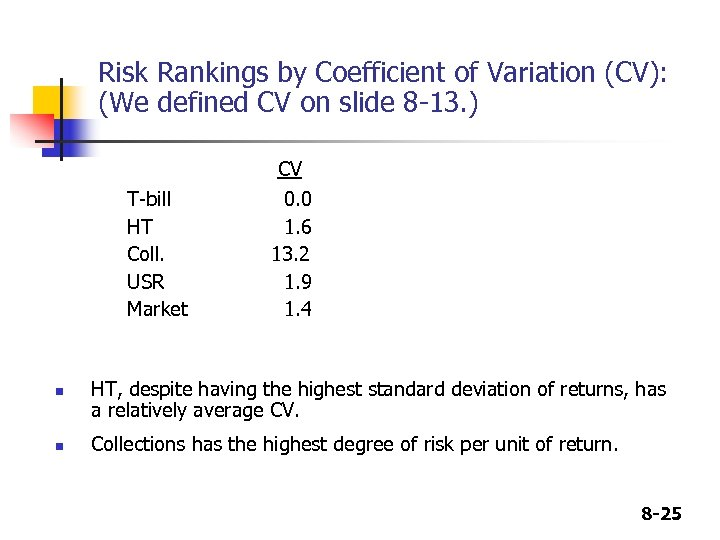Risk Rankings by Coefficient of Variation (CV): (We defined CV on slide 8 -13. ) T-bill HT Coll. USR Market n n CV 0. 0 1. 6 13. 2 1. 9 1. 4 HT, despite having the highest standard deviation of returns, has a relatively average CV. Collections has the highest degree of risk per unit of return. 8 -25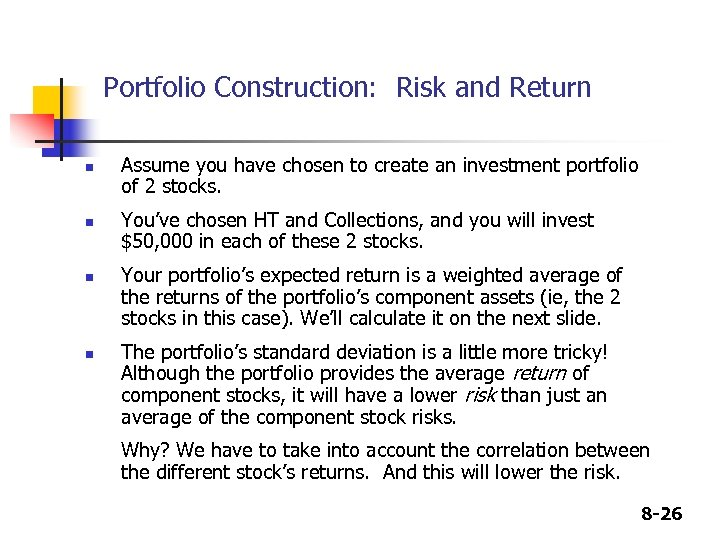Portfolio Construction: Risk and Return n n Assume you have chosen to create an investment portfolio of 2 stocks. You’ve chosen HT and Collections, and you will invest \$50, 000 in each of these 2 stocks. Your portfolio’s expected return is a weighted average of the returns of the portfolio’s component assets (ie, the 2 stocks in this case). We’ll calculate it on the next slide. The portfolio’s standard deviation is a little more tricky! Although the portfolio provides the average return of component stocks, it will have a lower risk than just an average of the component stock risks. Why? We have to take into account the correlation between the different stock’s returns. And this will lower the risk. 8 -26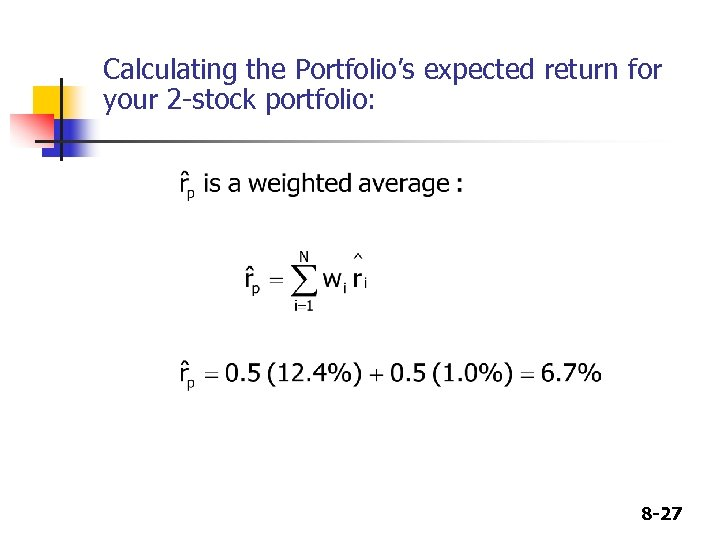Calculating the Portfolio’s expected return for your 2 -stock portfolio: 8 -27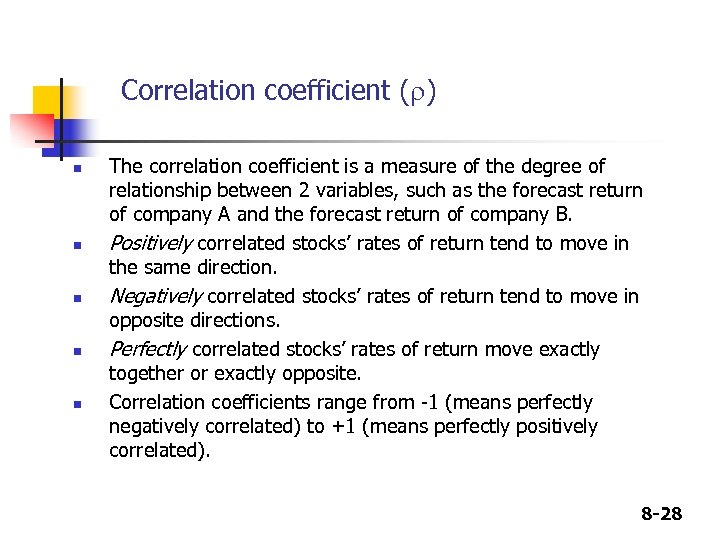Correlation coefficient (r) n n n The correlation coefficient is a measure of the degree of relationship between 2 variables, such as the forecast return of company A and the forecast return of company B. Positively correlated stocks’ rates of return tend to move in the same direction. Negatively correlated stocks’ rates of return tend to move in opposite directions. Perfectly correlated stocks’ rates of return move exactly together or exactly opposite. Correlation coefficients range from -1 (means perfectly negatively correlated) to +1 (means perfectly positively correlated). 8 -28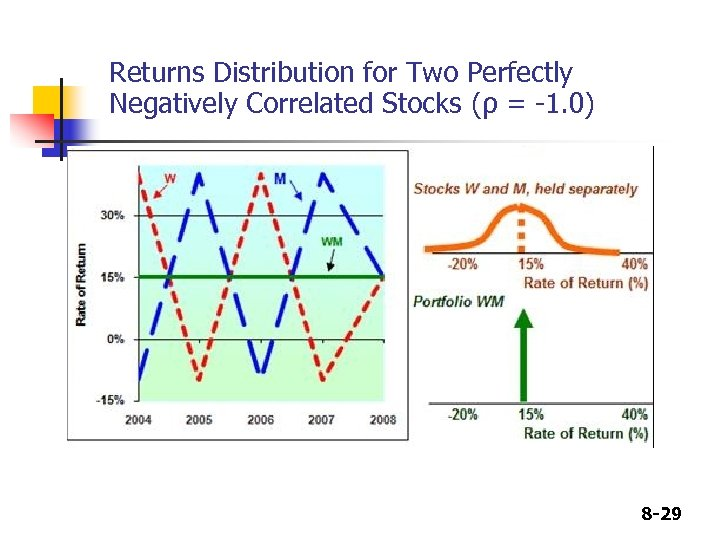Returns Distribution for Two Perfectly Negatively Correlated Stocks (ρ = -1. 0) 8 -29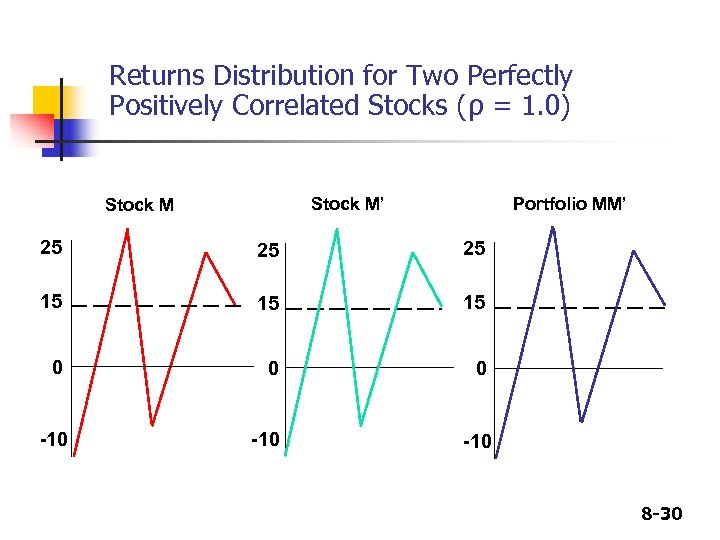Returns Distribution for Two Perfectly Positively Correlated Stocks (ρ = 1. 0) Stock M’ Stock M Portfolio MM’ 25 25 25 15 15 15 0 0 0 -10 -10 8 -30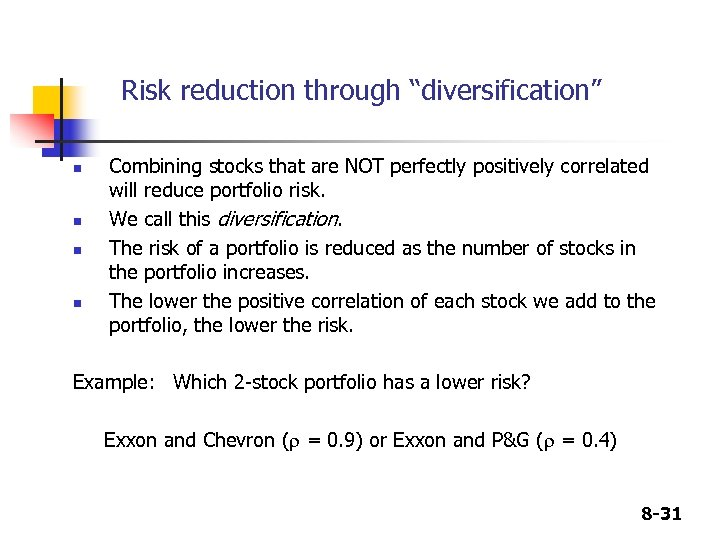Risk reduction through “diversification” n n Combining stocks that are NOT perfectly positively correlated will reduce portfolio risk. We call this diversification. The risk of a portfolio is reduced as the number of stocks in the portfolio increases. The lower the positive correlation of each stock we add to the portfolio, the lower the risk. Example: Which 2 -stock portfolio has a lower risk? Exxon and Chevron (r = 0. 9) or Exxon and P&G (r = 0. 4) 8 -31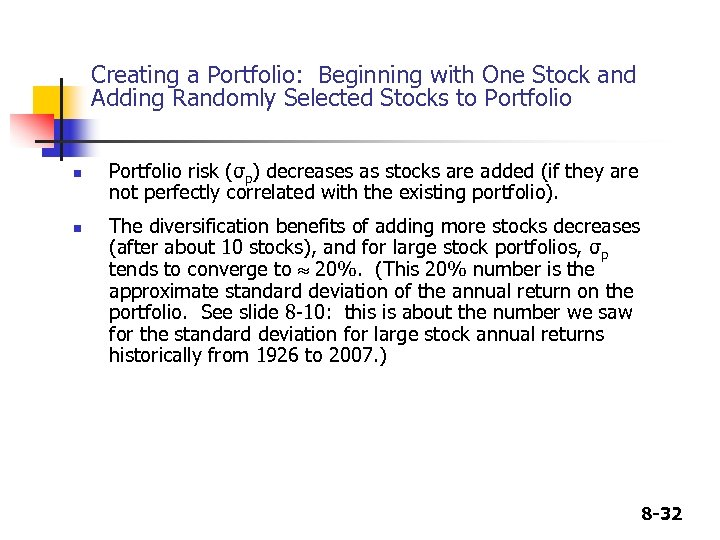Creating a Portfolio: Beginning with One Stock and Adding Randomly Selected Stocks to Portfolio n n Portfolio risk (σp) decreases as stocks are added (if they are not perfectly correlated with the existing portfolio). The diversification benefits of adding more stocks decreases (after about 10 stocks), and for large stock portfolios, σp tends to converge to 20%. (This 20% number is the approximate standard deviation of the annual return on the portfolio. See slide 8 -10: this is about the number we saw for the standard deviation for large stock annual returns historically from 1926 to 2007. ) 8 -32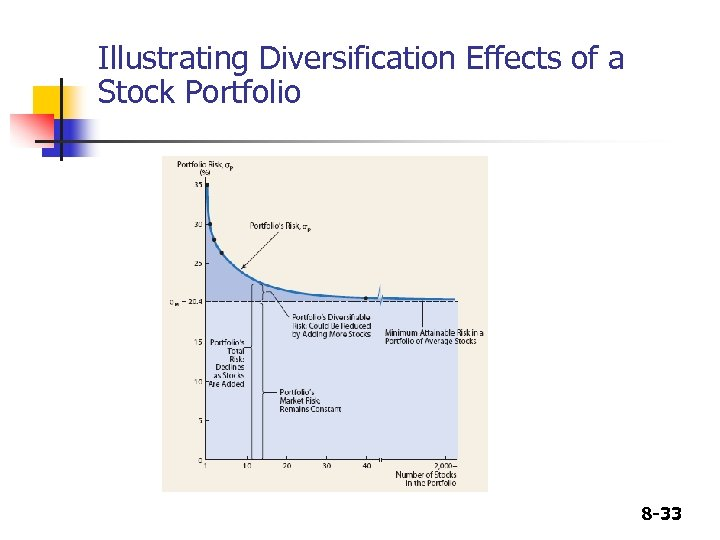Illustrating Diversification Effects of a Stock Portfolio 8 -33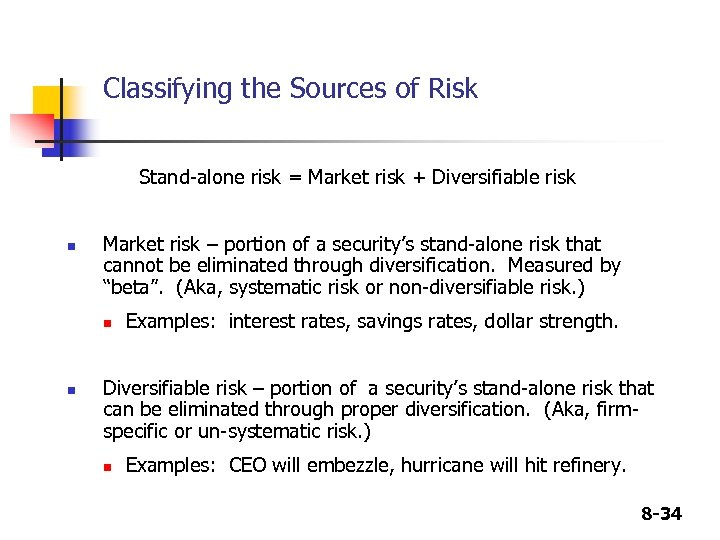Classifying the Sources of Risk Stand-alone risk = Market risk + Diversifiable risk n Market risk – portion of a security’s stand-alone risk that cannot be eliminated through diversification. Measured by “beta”. (Aka, systematic risk or non-diversifiable risk. ) n n Examples: interest rates, savings rates, dollar strength. Diversifiable risk – portion of a security’s stand-alone risk that can be eliminated through proper diversification. (Aka, firmspecific or un-systematic risk. ) n Examples: CEO will embezzle, hurricane will hit refinery. 8 -34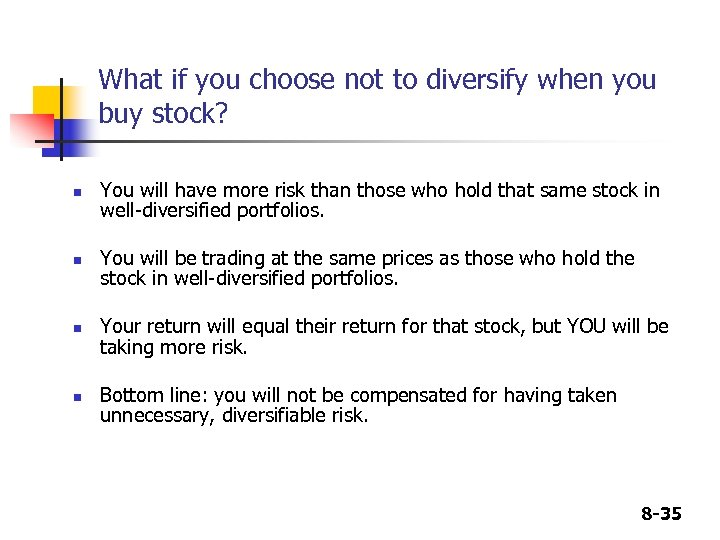What if you choose not to diversify when you buy stock? n n You will have more risk than those who hold that same stock in well-diversified portfolios. You will be trading at the same prices as those who hold the stock in well-diversified portfolios. Your return will equal their return for that stock, but YOU will be taking more risk. Bottom line: you will not be compensated for having taken unnecessary, diversifiable risk. 8 -35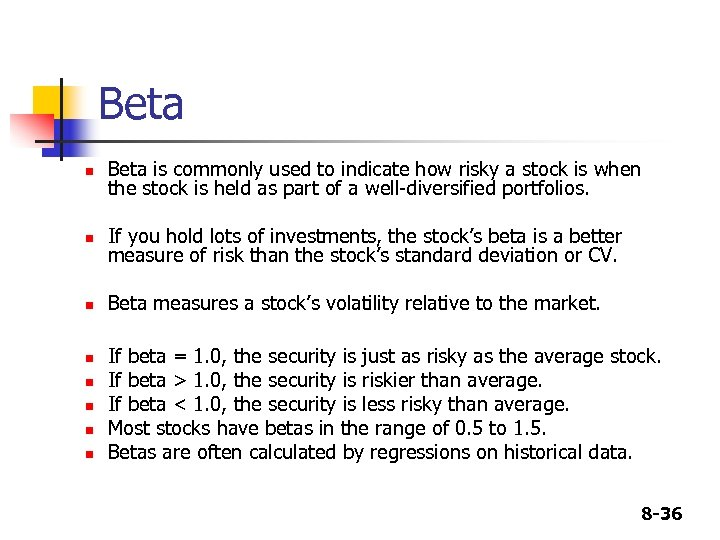Beta n Beta is commonly used to indicate how risky a stock is when the stock is held as part of a well-diversified portfolios. n If you hold lots of investments, the stock’s beta is a better measure of risk than the stock’s standard deviation or CV. n Beta measures a stock’s volatility relative to the market. n n n If beta = 1. 0, the security is just as risky as the average stock. If beta > 1. 0, the security is riskier than average. If beta < 1. 0, the security is less risky than average. Most stocks have betas in the range of 0. 5 to 1. 5. Betas are often calculated by regressions on historical data. 8 -36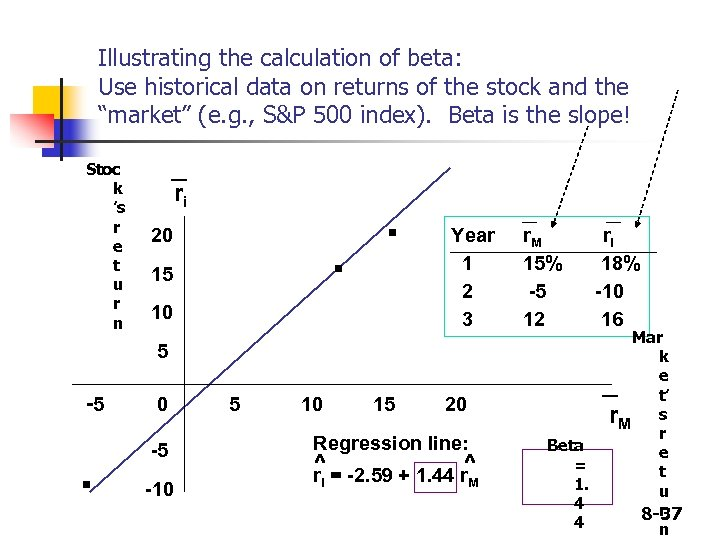Illustrating the calculation of beta: Use historical data on returns of the stock and the “market” (e. g. , S&P 500 index). Beta is the slope! Stoc k ’s r e t u r n _ ri . 20 15 . 10 Year 1 2 3 r. M 15% -5 12 5 -5 . 0 -5 -10 5 10 15 Mar k e t’ r. M s r e t u r 8 -37 n _ 20 Regression line: ^ = -2. 59 + 1. 44 r^ r i ri 18% -10 16 M Beta = 1. 4 4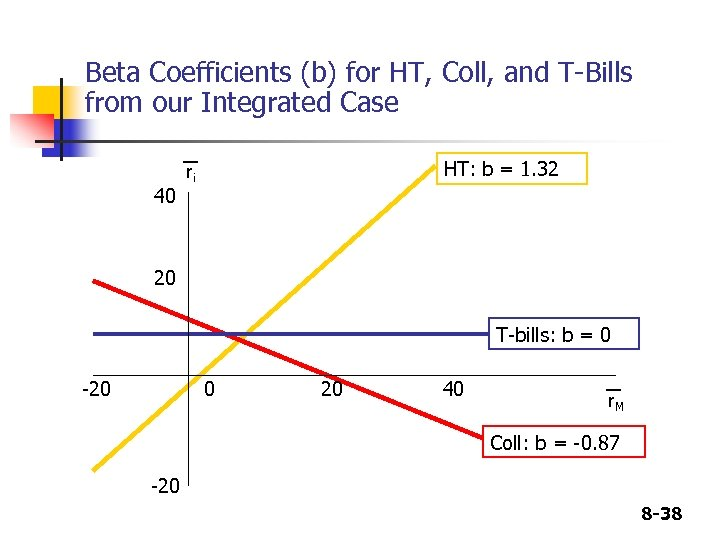Beta Coefficients (b) for HT, Coll, and T-Bills from our Integrated Case 40 HT: b = 1. 32 ri 20 T-bills: b = 0 -20 0 20 40 r. M Coll: b = -0. 87 -20 8 -38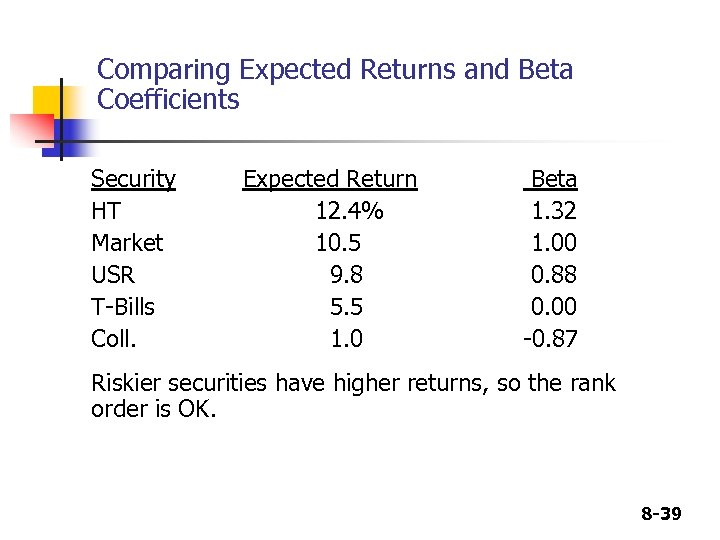Comparing Expected Returns and Beta Coefficients Security HT Market USR T-Bills Coll. Expected Return 12. 4% 10. 5 9. 8 5. 5 1. 0 Beta 1. 32 1. 00 0. 88 0. 00 -0. 87 Riskier securities have higher returns, so the rank order is OK. 8 -39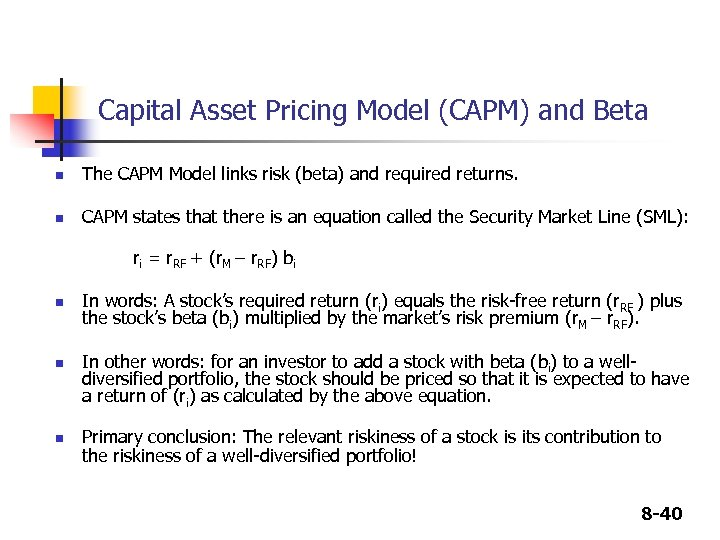Capital Asset Pricing Model (CAPM) and Beta n The CAPM Model links risk (beta) and required returns. n CAPM states that there is an equation called the Security Market Line (SML): ri = r. RF + (r. M – r. RF) bi n n n In words: A stock’s required return (ri) equals the risk-free return (r. RF ) plus the stock’s beta (bi) multiplied by the market’s risk premium (r. M – r. RF). In other words: for an investor to add a stock with beta (bi) to a welldiversified portfolio, the stock should be priced so that it is expected to have a return of (ri) as calculated by the above equation. Primary conclusion: The relevant riskiness of a stock is its contribution to the riskiness of a well-diversified portfolio! 8 -40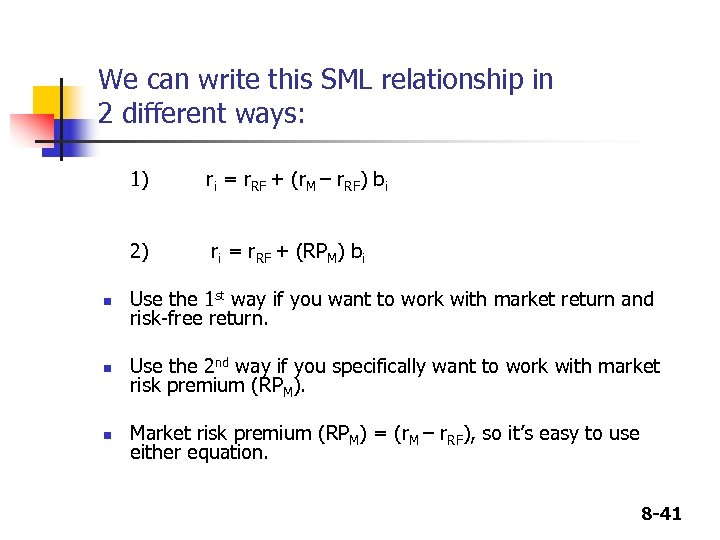We can write this SML relationship in 2 different ways: 1) 2) n n n ri = r. RF + (r. M – r. RF) bi ri = r. RF + (RPM) bi Use the 1 st way if you want to work with market return and risk-free return. Use the 2 nd way if you specifically want to work with market risk premium (RPM). Market risk premium (RPM) = (r. M – r. RF), so it’s easy to use either equation. 8 -41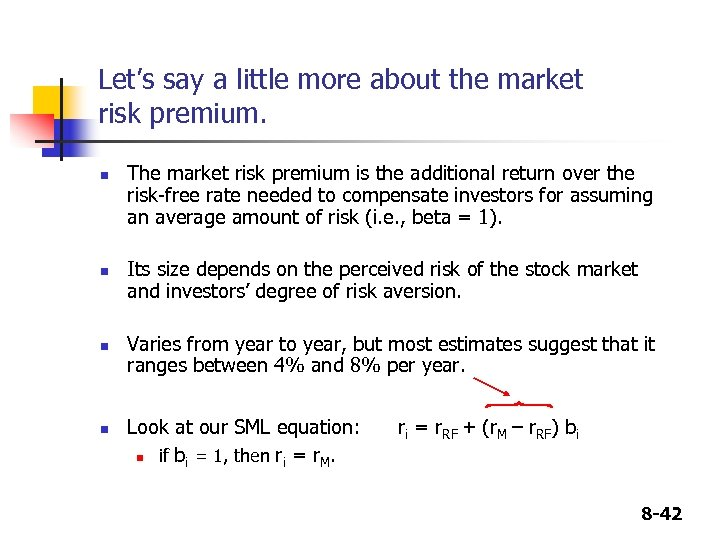Let’s say a little more about the market risk premium. n n The market risk premium is the additional return over the risk-free rate needed to compensate investors for assuming an average amount of risk (i. e. , beta = 1). Its size depends on the perceived risk of the stock market and investors’ degree of risk aversion. Varies from year to year, but most estimates suggest that it ranges between 4% and 8% per year. Look at our SML equation: n if bi = 1, then ri = r. M. ri = r. RF + (r. M – r. RF) bi 8 -42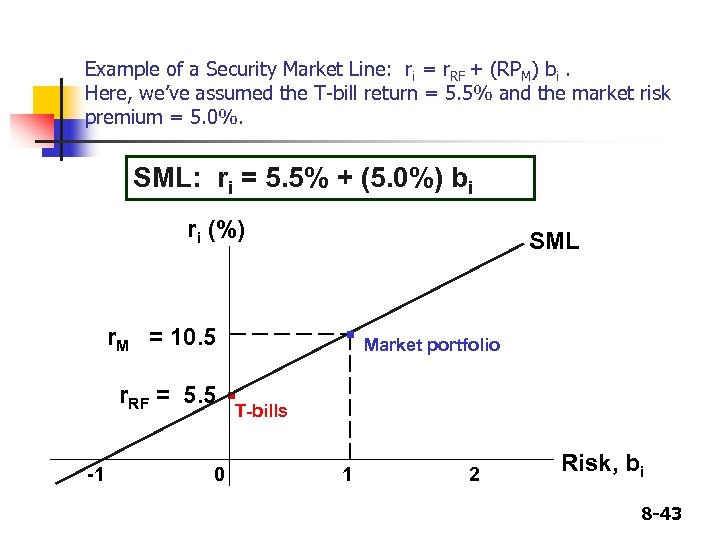Example of a Security Market Line: ri = r. RF + (RPM) bi. Here, we’ve assumed the T-bill return = 5. 5% and the market risk premium = 5. 0%. SML: ri = 5. 5% + (5. 0%) bi ri (%) r. M = 10. 5 r. RF = 5. 5 -1 SML . . 0 Market portfolio T-bills 1 2 Risk, bi 8 -43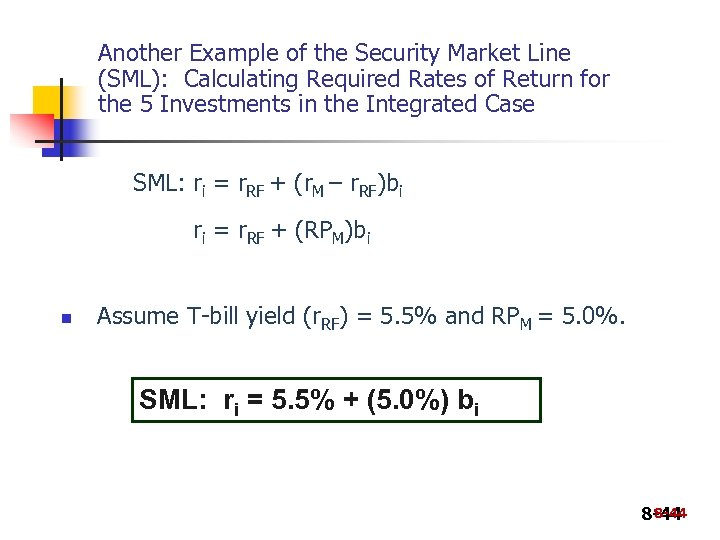Another Example of the Security Market Line (SML): Calculating Required Rates of Return for the 5 Investments in the Integrated Case SML: ri = r. RF + (r. M – r. RF)bi ri = r. RF + (RPM)bi n Assume T-bill yield (r. RF) = 5. 5% and RPM = 5. 0%. SML: ri = 5. 5% + (5. 0%) bi 8 -44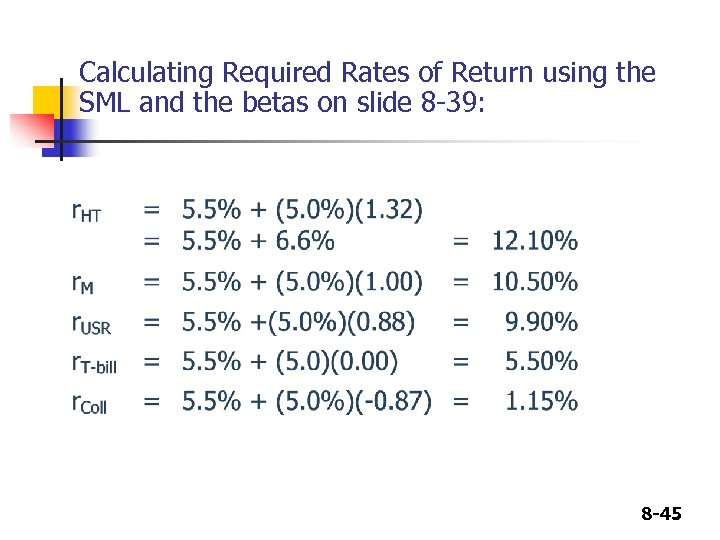Calculating Required Rates of Return using the SML and the betas on slide 8 -39: 8 -45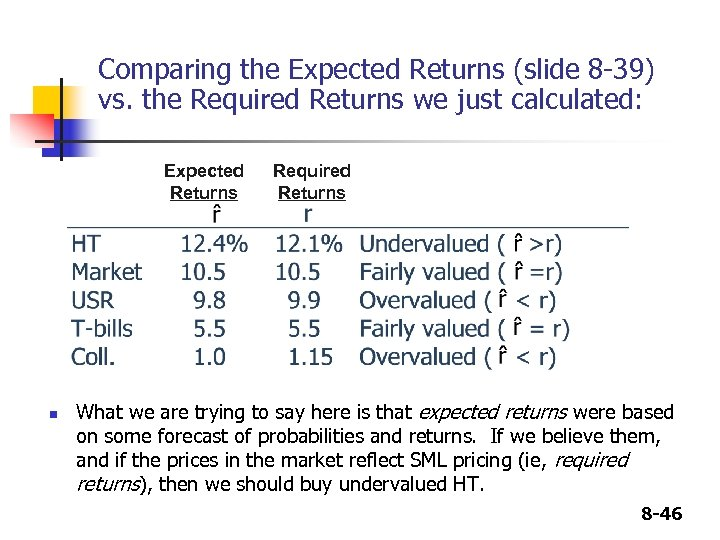Comparing the Expected Returns (slide 8 -39) vs. the Required Returns we just calculated: Expected Returns n Required Returns What we are trying to say here is that expected returns were based on some forecast of probabilities and returns. If we believe them, and if the prices in the market reflect SML pricing (ie, required returns), then we should buy undervalued HT. 8 -46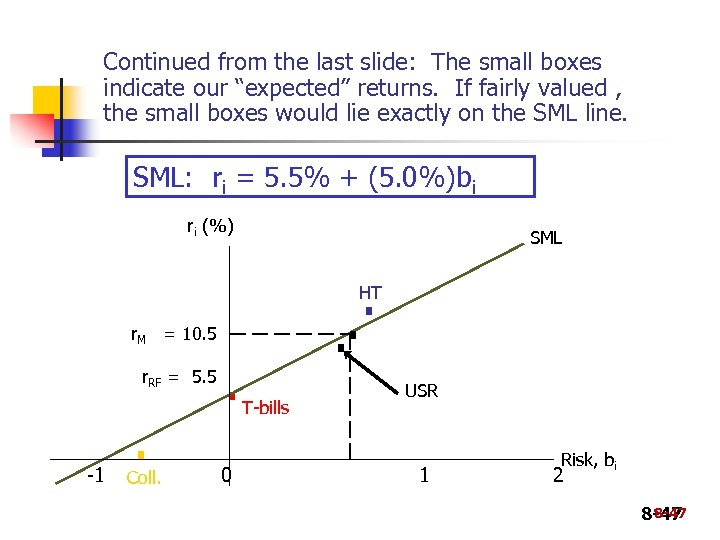Continued from the last slide: The small boxes indicate our “expected” returns. If fairly valued , the small boxes would lie exactly on the SML line. SML: ri = 5. 5% + (5. 0%)bi ri (%) SML . . . HT r. M = 10. 5 r. RF = 5. 5 -1 . Coll. . 0 T-bills USR 1 Risk, bi 2 8 -47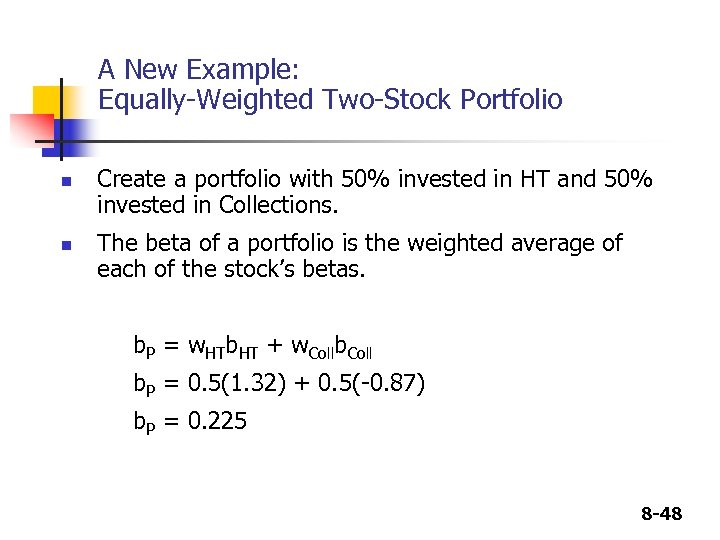A New Example: Equally-Weighted Two-Stock Portfolio n n Create a portfolio with 50% invested in HT and 50% invested in Collections. The beta of a portfolio is the weighted average of each of the stock’s betas. b. P = w. HTb. HT + w. Collb. Coll b. P = 0. 5(1. 32) + 0. 5(-0. 87) b. P = 0. 225 8 -48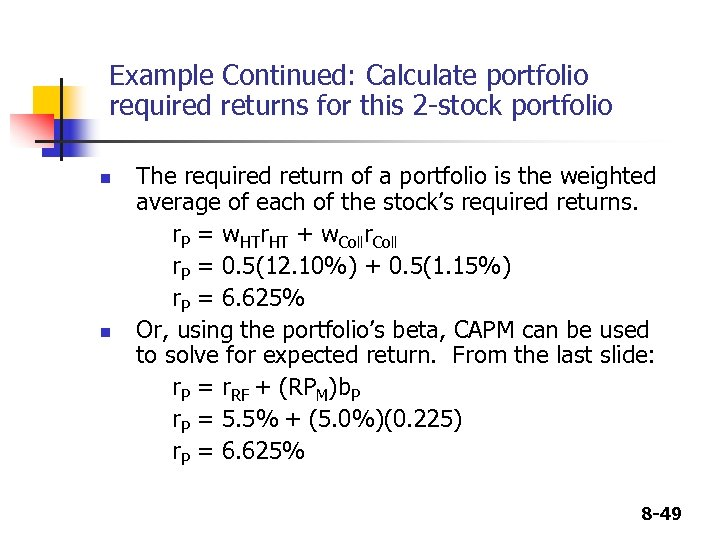Example Continued: Calculate portfolio required returns for this 2 -stock portfolio n n The required return of a portfolio is the weighted average of each of the stock’s required returns. r. P = w. HTr. HT + w. Collr. Coll r. P = 0. 5(12. 10%) + 0. 5(1. 15%) r. P = 6. 625% Or, using the portfolio’s beta, CAPM can be used to solve for expected return. From the last slide: r. P = r. RF + (RPM)b. P r. P = 5. 5% + (5. 0%)(0. 225) r. P = 6. 625% 8 -49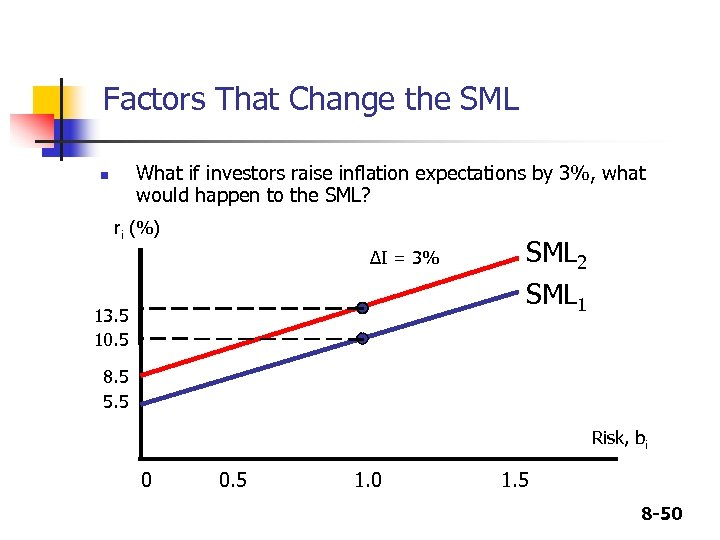Factors That Change the SML What if investors raise inflation expectations by 3%, what would happen to the SML? n ri (%) ΔI = 3% 13. 5 10. 5 SML 2 SML 1 8. 5 5. 5 Risk, bi 0 0. 5 1. 0 1. 5 8 -50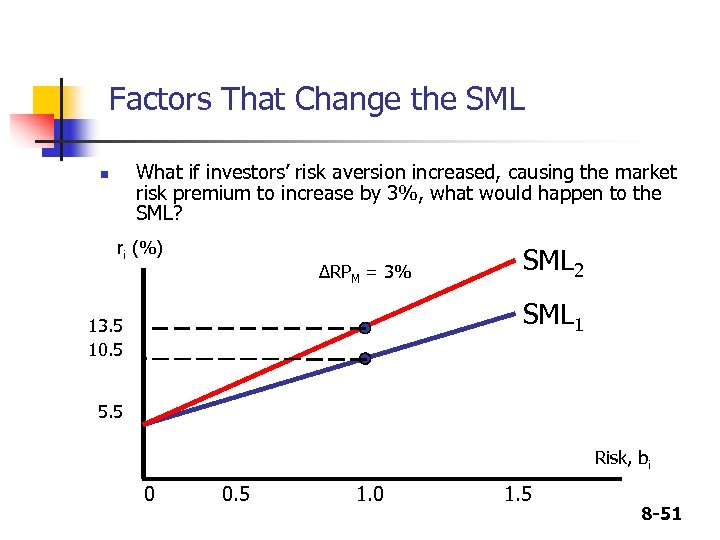Factors That Change the SML What if investors’ risk aversion increased, causing the market risk premium to increase by 3%, what would happen to the SML? n ri (%) ΔRPM = 3% SML 2 SML 1 13. 5 10. 5 5. 5 Risk, bi 0 0. 5 1. 0 1. 5 8 -51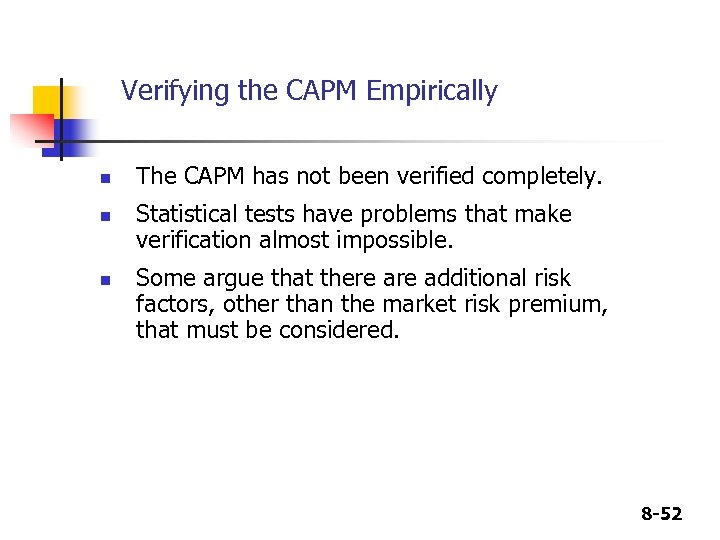Verifying the CAPM Empirically n n n The CAPM has not been verified completely. Statistical tests have problems that make verification almost impossible. Some argue that there additional risk factors, other than the market risk premium, that must be considered. 8 -52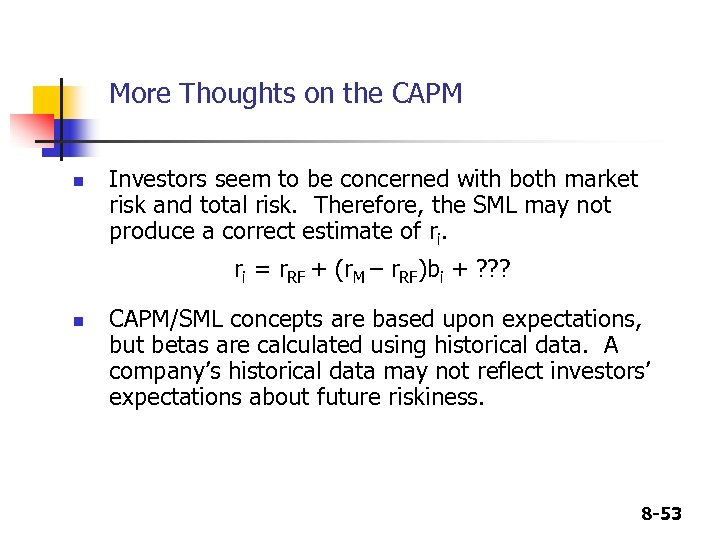More Thoughts on the CAPM n Investors seem to be concerned with both market risk and total risk. Therefore, the SML may not produce a correct estimate of ri. ri = r. RF + (r. M – r. RF)bi + ? ? ? n CAPM/SML concepts are based upon expectations, but betas are calculated using historical data. A company’s historical data may not reflect investors’ expectations about future riskiness. 8 -53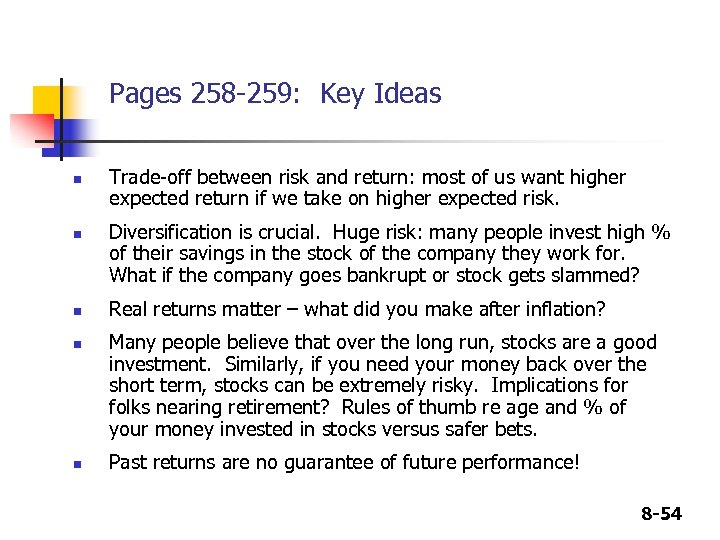Pages 258 -259: Key Ideas n n n Trade-off between risk and return: most of us want higher expected return if we take on higher expected risk. Diversification is crucial. Huge risk: many people invest high % of their savings in the stock of the company they work for. What if the company goes bankrupt or stock gets slammed? Real returns matter – what did you make after inflation? Many people believe that over the long run, stocks are a good investment. Similarly, if you need your money back over the short term, stocks can be extremely risky. Implications for folks nearing retirement? Rules of thumb re age and % of your money invested in stocks versus safer bets. Past returns are no guarantee of future performance! 8 -54